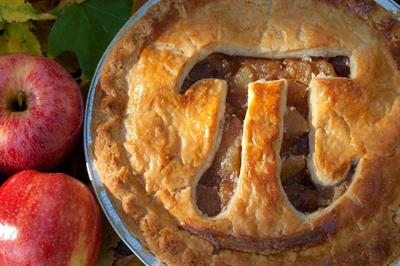Search - School / College / Institute

# International Pi DayThe number π (/paɪ/) is a mathematical constant. Originally defined as the ratio of a circle's circumference to its diameter, it presently has various equivalent definitions and appears in many formulas in all various areas of mathematics and physics. It is approximately equal to 3.14159. It has been represented by the Greek letter "π" since the mid-18th century. It is also sometimes spelled out as "pi". March 14 is celebrated every year as International Pi Day. The exact value of Pi has so far been calculated upto 22 trillion digits, although it is believed to go on indefinitely. Some of the amazing facts about Pi are as follows:

1. Birth of Albert Einstein

March 14 which is celebrated as Pi Day, also marks the birthday of the great physicist Albert Einstein. He was born on March 14, 1879.

2. Pi Day or Pie Day

People around the globe indulge in celebrating the Pi Day by grabbing a slice of Pie. That sounds cool, doesn’t it?

3. Trillion Digit Value

The exact ratio hasn’t been calculated yet. Pi enthusiast Peter Trueb's computer calculated pi to 22,459,157,718,361 digits. In order to make this possible, he had to build a computer with 24 hard drives. Each of it contains six terabytes of memory.

4. Value of Pi by heart

Suresh Kumar Sharma of India, has set the record for memorizing the value of Pi upto 70,030 digits in October 2015. It took him 17 hours and 14 minutes to rhyme off what he learnt.

5. Digits

There are no occurences of 123456 in the first million digits of pi. But the first six digits, 314159, are present in that particular order at least six times in the first 10 million decimal places.

6. History of Pi

At first the Babylonians calculated pi approximately 4000 years ago. Later an Egyptian scribe calculated a circle’s area giving it approximate value of 3.16. Around 287 B.C. Mathematician Archimedes used the Pythagorean Theorem to calculate Pi. Further Chinese mathematician Zu Chongzhi, also calculated it to 3.14159292. It was in 1700s that the Greek letter π began to be used to represent pi.

By: Anuja Arora

Source: http://www.cbc.ca/news/technology/pi-day-facts-1.4572534### Future Bright Program

Enhance Your Skills With Our ExpertsInteractive School Platform